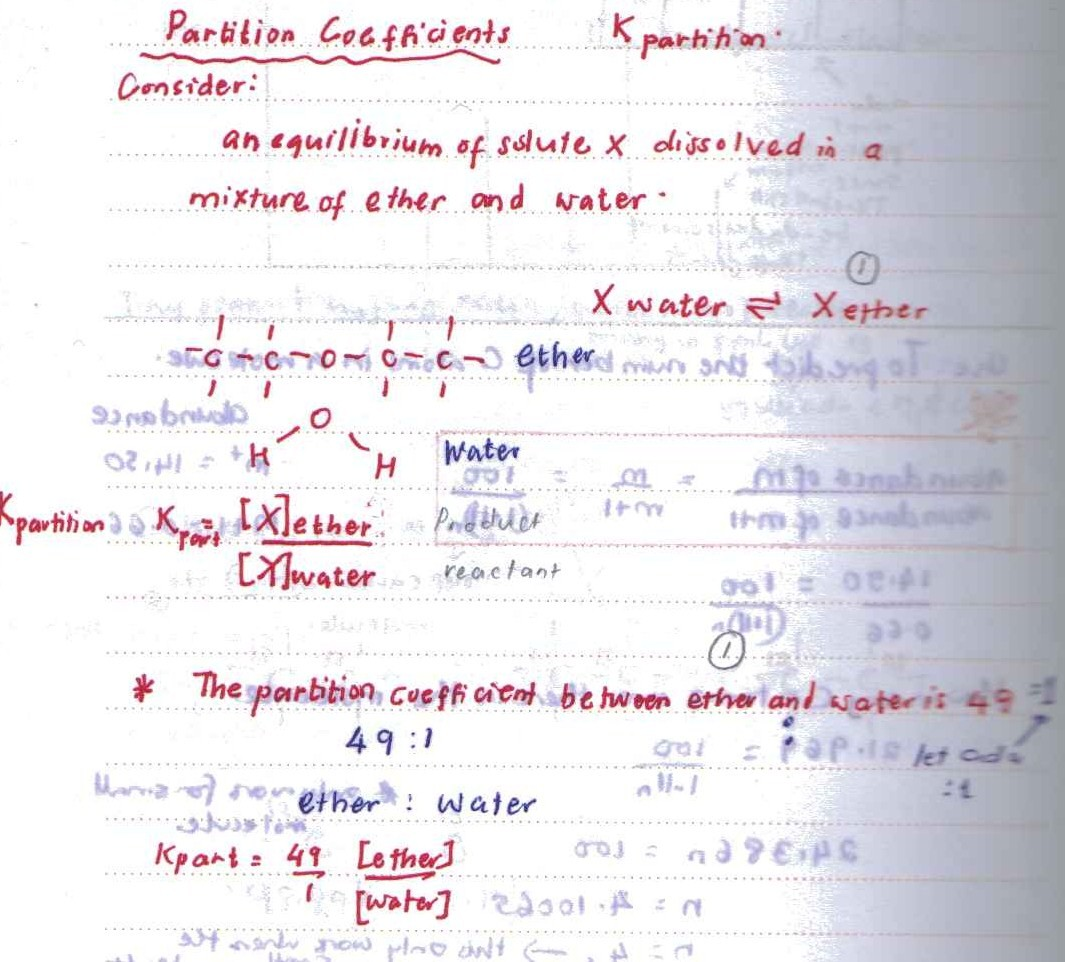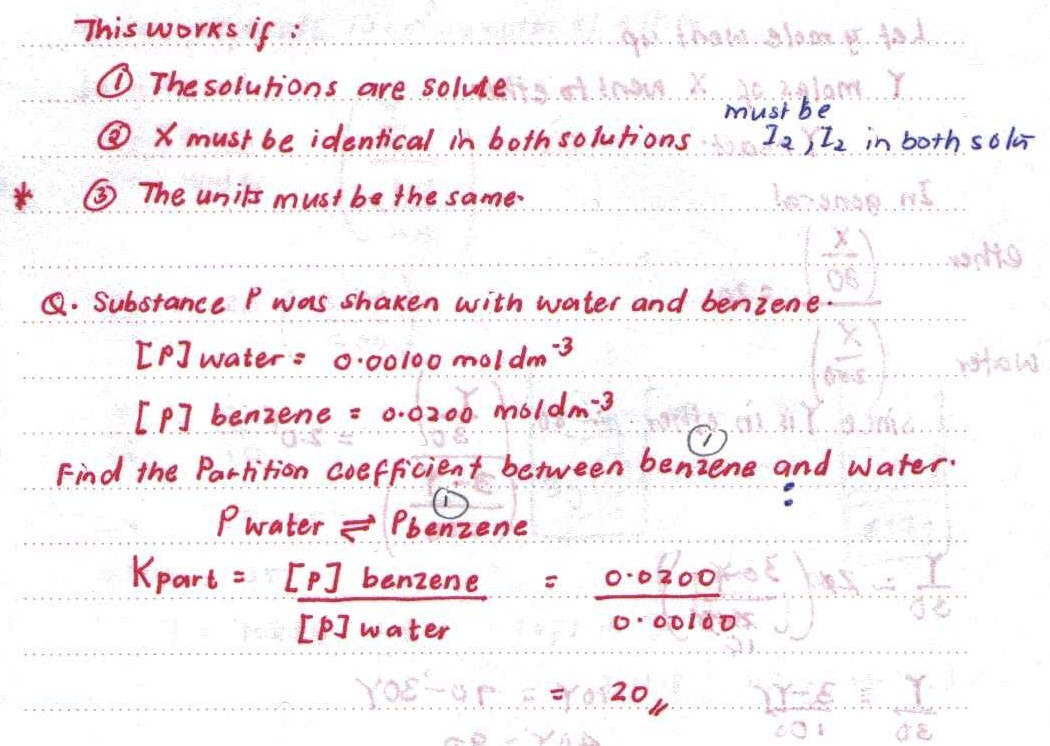# Partition Coefficient

Partition is when two liquids or solvent are having the division from mixing. This works when the two liquid are immicible. This partition occur in dynamic equilibrium where a substance is dissolving. Partition coefficient equation is as follows:

Kpartition = [X(liquid 1)]/[X(liquid 2)] this equation of Kpartition has no units and only temperature can affect the reaction.

Why does the Kpartition has no unit?

This is due to the same units for both concentration and they both cancelled in the equation.When two phases are involved, other than the same phases and units, the units at the end of the equation will depend on the units used for each phases.

### Reference

1. Harword R(2005)Application Book Cambridge (80-82)